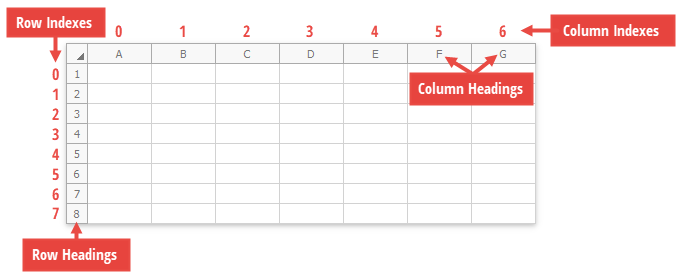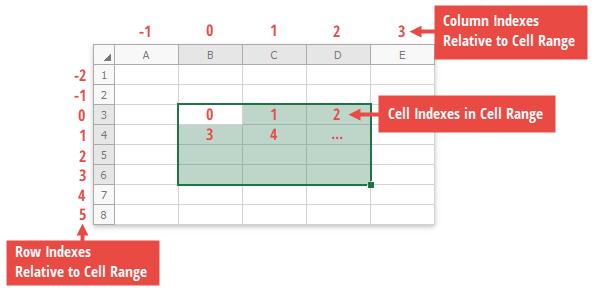21.2
21.1
20.2
20.1
19.2
19.1
18.2
18.1
17.2
.NET Framework 4.5.2+
.NET Framework 4.5.2+
.NET Standard 2.0+

# How to: Access a Cell in a Worksheet

This example demonstrates several ways to access individual cells.

• By row and column indexes of a cell via the Worksheet.Item property.
• By a cell reference (for example, A1, B2, etc.) or by row and column indexes from the worksheet’s collection of cells. Use the CellCollection.Item property.
• By a column index or column heading from the specified row. Use the Row.Item property.

By a row index or row heading from the specified column. Use the Column.Item property.

See the How to: Access a Row or Column example to learn how to get an individual row or column.

• By a cell index or by row and column indexes from the specified range of cells. Use the CellRange.Item property. See the How to: Access a Range of Cells document to learn how to access an individual range of cells in a worksheet.
``````using DevExpress.Spreadsheet;
// ...

Worksheet worksheet = workbook.Worksheets;

Cell cellA1 = worksheet[0, 0]; // Cell A1

Cell cellB2 = worksheet.Cells["B2"]; // Cell B2
Cell cellC2 = worksheet.Cells[1, 2]; // Cell C2

Cell cellD3 = worksheet.Cells[2, 3]; // Cell D3
Cell cellE4 = worksheet.Rows["E"]; // Cell E4
Cell cellF4 = worksheet.Rows["4"]; // Cell F4

Cell cellG5 = worksheet.Columns; // Cell G5
Cell cellH6 = worksheet.Columns["H"]; // Cell H6
Cell cellI7 = worksheet.Columns["I"]["7"]; // Cell I7

// Access a cell from the range of cells.
Cell cellB5 = worksheet.Range["B3:D6"]; // Cell B5
Cell cellD6 = worksheet.Range["B3:D6"][3, 2]; // Cell D6
``````

The following images demonstrate how rows and columns are indexed in a worksheet and in a cell range.

Row and Column Indexes and Names in Worksheet Cell, Row and Column Indexes in a Cell Range